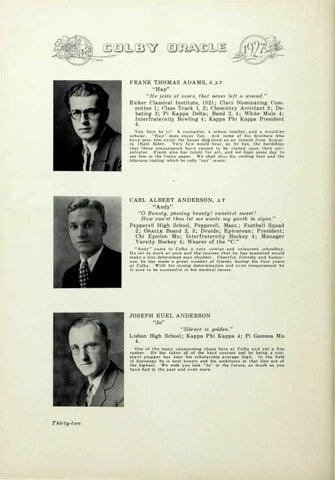F RA N K T H O M A S A D A M S , K � P "Hap"

" H e jests a t soars, t ha t neve 1· left a w<>und." R icker C l a ssical Institute, 1 9 2 1 ; C l a Nomin ating Committee 1; �l ass T rack 1, 2; Chem i try A i tant 2 ; De­ bating 3; Pi Kappa Delta; Band 3 , 4 ; Wh ite M u le 4; I nterfrate rn i ty Bowling 4; Kappa Phi Kappa President 4. Yes. here h e i ! A y o u n gster, a sc hool teacher, a n d a w o u l d - b e s c h o la r . "Hap" does enjoy fun. A k s o m e of h i brothers w h o h a v e e e n h i m e n t e r t h e h o u s e d i g u i ed a s a n i n mate from Aug u s ­ ta ( E a s t Side ) . Very f e w w o u l d b e a r , a s h e has, t h e h a r d s h i p s that t h ese a m u se m e n t s h a v e cau sed t o be v i s i ted upon t h e i r p e r ­ petrator. Fra n k a l s o h a s talent for a rt , a n d we hope some day to see h i m i n t h e fun n y paper. W e shall m i hi m i l i ng face a n d the h i larious tooting which he calls "sax·· m usic .

CARL A LBERT AN DERSON, � T

'�� , ;t. . ·

. ··

.

). . . .

.

. \ • .� •

'

··

"O

"Andy"

Beauty, passing beauty ! sweetest swee t ! How oan'st t hau let me was t e nvy yonth in signs."

Pepperell H igh School , Pepperell , Mas . ; Footba l l Squad 2; O RACLE Board 2, 3; D r u i d s ; E p icurean; P resident; Chi E psilon Mu; In terfraternity Hockey 4 ; Manage r Vars ity Hockey 4; Wearer of t h e " C . " " A n d y " c a m e to Colby a very u n w i se a n d u n learned c h oo l bo y . H e . e t to w o r k a t o n c e a n d the c o u rses t h a t h e h a s m astered w o u l d m a k e a l e s s deter m i n ed m a n s h u dder. C h ee r f u l , f r i e n d l y a n d h u m o r ­ o u s , h e has made a g r e a t n u m b e r of friend d u ri n g b i s f o u r y e a r s a t Colby. W i t h h i s s t ro n g deter m in a t i o n a n d e v e n t e m p e ra m e n t b e i sure t o be s u cces s f u l i n b i s m e d i c a l career.

JOSEPH RUEL A

DERSON

"Jo"

"Sile nce is golden." L isbon High School ; Kappa P h i Kappa 4; Pi Gamma M u 4. One o f t h e m a n y u n a s s u m i n g chaps here a t Colby a n d yet a fi ne ra n k e r . He has taken all of the hard c o u rses and by being a c o n ­ s t a n t p l u gger has kept h i s s c h o l a r s h i p average h i g h . In t h e field of Sociology he i s best k n o w n and his a m b i t i o n s in that l i n e a r e of t h e h ig h es t . W e wish y o u luck "Jo" in the f u t u re, a s m u c h a s you have b a d in t h e past a n d even more.

T hfrty-two